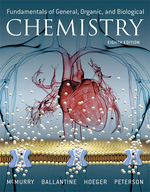×
Get Full Access to Fundamentals Of General, Organic, And Biological Chemistry (Mastering Chemistry) - 8 Edition - Chapter 7.6 - Problem 7.10
Get Full Access to Fundamentals Of General, Organic, And Biological Chemistry (Mastering Chemistry) - 8 Edition - Chapter 7.6 - Problem 7.10

×ISBN: 9780134015187 2044

## Solution for problem 7.10 Chapter 7.6

Fundamentals of General, Organic, and Biological Chemistry (Mastering Chemistry) | 8th Edition

• Textbook Solutions
• 2901 Step-by-step solutions solved by professors and subject experts
• Get 24/7 help from StudySoup virtual teaching assistantsFundamentals of General, Organic, and Biological Chemistry (Mastering Chemistry) | 8th Edition

4 5 1 265 Reviews
24
4
Problem 7.10

Ammonia is synthesized industrially by reaction of nitrogen and hydrogen according to the equation $$3 \mathrm{H}_{2}(g)+\mathrm{N}_{2}(g) \longrightarrow 2 \mathrm{NH}_{3}(g)$$. The free-energy change for this reaction is $$\Delta G=-16 \mathrm{~kJ} / \mathrm{mol}$$, yet this reaction does not readily occur at room temperature. List three ways to increase the rate of this reaction.

Text Transcription:

3 H_2(g)+N_2(g) longrightarrow 2 NH_3(g)

Delta G=-16 ~kJ/mol

Step-by-Step Solution:
Step 1 of 3

( 8/26 – 8/30 ) Organic Chemistry 8/26 Introduction to Organic Chemistry  Organic Chemistry is the study of the structure, properties, behavior, and the reaction of molecules containing the element carbon o Molecules containing carbon are called Organic Compounds  Organic Compounds can also contain hydrogen, nitrogen, oxygen, sulfur, phosphorus, and the halogens (Group 7A).  Any molecule that does not contain the element carbon is called an Inorganic Compound Covalent Bonds  Covalent Bonds are bonds between elements that only nonmetal and they bond by sharing electrons  Most elements in organic compounds are nonmetal; therefore, contain covalent bonds 8/28 Stability in Atoms  Any atoms whose Valence Shell is filled is considered stable o The Valence Shell is the outer-most shell around an atom  Some atoms have more than one shell, so the Valence Shell is the furthest one from the nucleus o This can either be accomplished by having 2 electrons in the Valence Shell (H or He) or 8 electrons (the octet rule)  The Octet Rule explains that an atom must have 8 electrons in the outer shell, but there are exceptions ( 8/26 – 8/30 )  Diatonic Fluorine is more stable than a single Fluorine molecule because the outer shell is filled  Less stable elements have a higher energy  When the free

Step 2 of 3

Step 3 of 3

##### ISBN: 9780134015187

This textbook survival guide was created for the textbook: Fundamentals of General, Organic, and Biological Chemistry (Mastering Chemistry), edition: 8. This full solution covers the following key subjects: . This expansive textbook survival guide covers 281 chapters, and 240 solutions. The full step-by-step solution to problem: 7.10 from chapter: 7.6 was answered by Aimee Notetaker, our top Chemistry solution expert on 04/25/22, 03:57PM. Fundamentals of General, Organic, and Biological Chemistry (Mastering Chemistry) was written by Aimee Notetaker and is associated to the ISBN: 9780134015187. Since the solution to 7.10 from 7.6 chapter was answered, more than 203 students have viewed the full step-by-step answer. The answer to “?Ammonia is synthesized industrially by reaction of nitrogen and hydrogen according to the equation $$3 \mathrm{H}_{2}(g)+\mathrm{N}_{2}(g) \longrightarrow 2 \mathrm{NH}_{3}(g)$$. The free-energy change for this reaction is $$\Delta G=-16 \mathrm{~kJ} / \mathrm{mol}$$, yet this reaction does not readily occur at room temperature. List three ways to increase the rate of this reaction.Text Transcription:3 H_2(g)+N_2(g) longrightarrow 2 NH_3(g)Delta G=-16 ~kJ/mol” is broken down into a number of easy to follow steps, and 58 words.

Unlock Textbook Solution